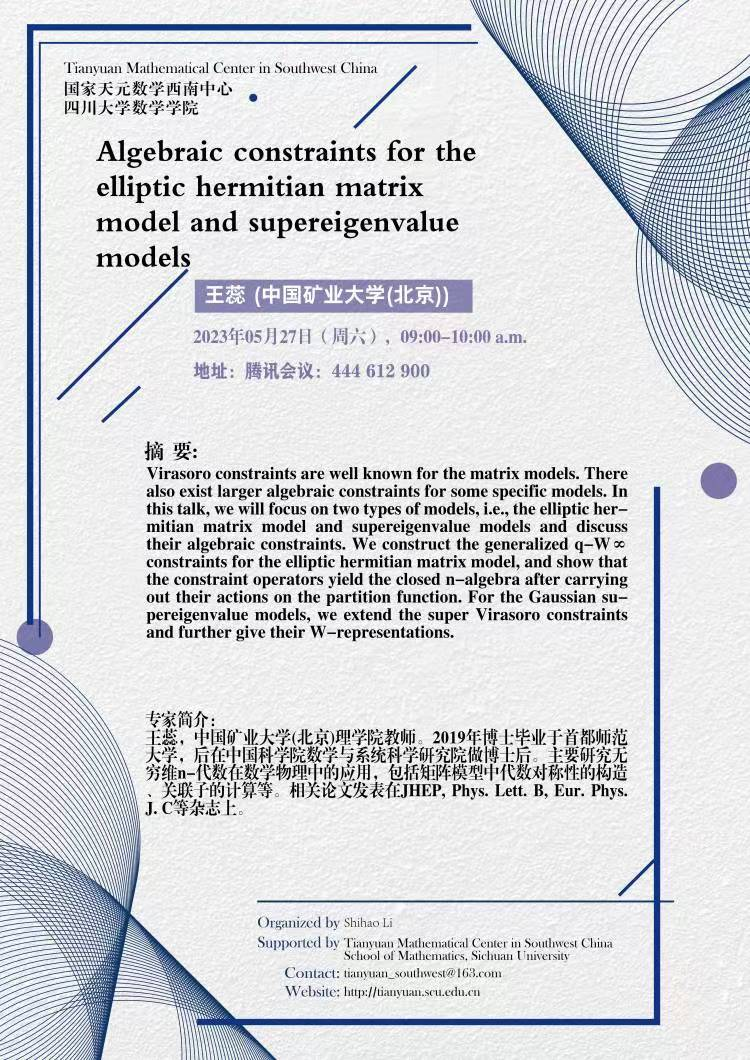Algebraic constraints for the elliptic hermitian matrix model and supereigenvalue models

Virasoro constraints are well known for the matrix models. There also exist larger algebraic constraints for some specific models. In this talk, we will focus on two types of models, i.e., the elliptic hermitian matrix model and supereigenvalue models and discuss their algebraic constraints. We construct the generalized q-W∞ constraints for the elliptic hermitian matrix model, and show that the constraint operators yield the closed n-algebra after carrying out their actions on the partition function. For the Gaussian supereigenvalue models, we extend the super Virasoro constraints and further give their W-representations.